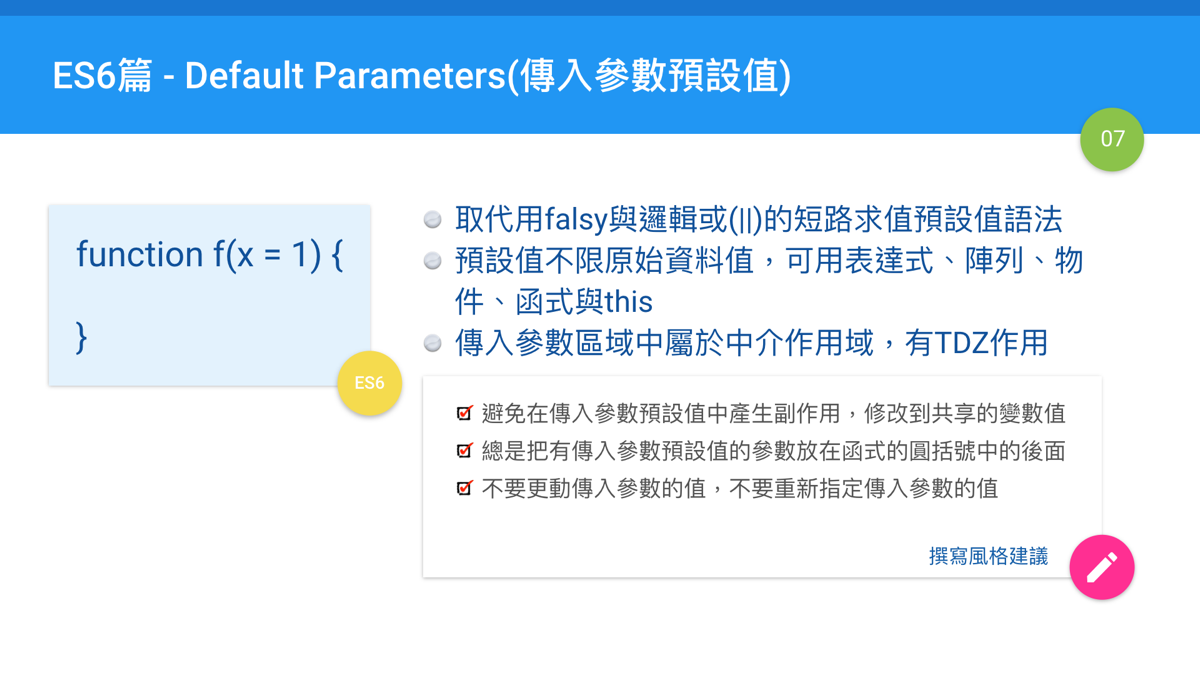#2017 iT 邦幫忙鐵人賽
DAY 7
0
Modern Web

## Day 07: ES6篇 - Default Parameters(傳入參數預設值)• parameters: 指的是在函式的那些傳入參數名稱的定義。我們在文章中會以"傳入參數定義名稱"來說明。
• arguments: 指的是當函式被呼叫時，傳入到函式中真正的那些值。我們在文章中會以"實際傳入參數值"來說明。

## 之前的傳入參數預設值怎麼作

``````//用邏輯或符號(||)
const link = function (point, url) {
let point = point || 10
let url = url || 'http://google.com'
...
}

//另一種設定的方式，typeof是回傳類型的字串值
const link = function (point, url) {
let point = typeof point !== 'undefined' ? point : 10
let url = typeof url !== 'undefined' ? url : 'http://google.com'
...
}
``````

``````console.log('foo' || 'bar') // 'foo'
console.log(false || 'bar') // 'bar'
``````

falsy的概念是另一個重要的JavaScript概念，Douglas Crockford大師主張使用"truthy"與"falsy"來描述資料類型的值(在JavaScript: The Good Parts這本書中有這一段)。也就是說，像上面說講的那些會轉換為布林值的false值的資料類型的值，通稱為"falsy"(字典裡是沒這個字詞，意思是"false的")，你可以把它當成是"false家族成員"，大概的定義如下:

"falsy"包含了0, -0, null, NaN, undefined, ''(空白字串)，當然也一定包含了false值

``````if (isValid) {
// ...stuff...
}

if (!name) {
// ...stuff...
}

if (!collection.length) {
// ...stuff...
}
``````

• 空物件({})與空陣列([])都不是falsy成員之一，實際上對於物件類型的值完全無法判斷
• 如果預設值可以是0或''(空白字串)，甚至可以是null，這種方式判斷不出來，要額外再作判斷

## ES6的傳入參數預設值

``````const link = function (point = 10, url = 'http://google.com') {
//...
}
``````

ES6的傳入參數預設值的設計，與許多程式語言中類似的設計都有點不太一樣。以下分別把重點的不同之處列出來。

### 傳入參數預設值裡可以用表達式

``````function foo(a = 0, b = a+100) {
console.log(a+b)
}

foo() // 100
foo(1)  // 102
foo(1, 2) // 3
``````

``````function go(x) {
return x + 10
}

function foo(a = 1, b = go(a)) {
console.log(a+b)
}

foo()  // 30
foo(1)  // 12
foo(1, 2) // 3
``````

### 傳入參數預設值的求值是每個函式呼叫獨立的(Evaluated at call time)

``````function foo(x = []) {
x.push(1)
console.log(x)
}

foo() // 
foo() // 
foo() // 
``````

### 傳入參數預設值會隱蔽外在的作用域

``````let x = 1

function foo(x, y = x) {
console.log(y)
}

foo(2) // 2, not 1!
``````

``````function foo(x = y, y = 1) {
console.log(y)
}

foo(1) // 這不會有錯誤
foo(undefined, 1) // 錯誤 ReferenceError: y is not defined
foo() // 錯誤 ReferenceError: y is not defined
``````

### 傳入參數預設值使用物件、其他函式與this時

``````function foo(x = this, y = this.value) {
console.log(x)
console.log(y)
}

foo()
foo({value: '= =+'})
foo.call({value: '^^y~'})
``````

``````let x = 1

function foo(a = 1, b = function(){ x = 2 }){
let x = 3
b()
console.log(x)
}

foo()

console.log(x)
``````

• babel編譯器: 2與1
• Traceur/Closure Compiler: 3與2
• Firefox(v50): 2與1
• Edge(v38): 3與2

3與2的答案是讓b傳入參數的`x=2`執行出來，但因為受到中介作用域的影響，因此干擾不到函式中的原本區塊中的作用域，但會影響到全域中的x變數。

2與1的答案則是倒過來，只會影響到函式中的區塊，對全域沒有影響。

### 必要的傳入參數

``````//方式一
function func1(x){
if(x === undefined) throw new Error('Missing parameter')

//...
}

//方式二
function throwIfMissing() {
throw new Error('Missing parameter');
}

function func2(x = throwIfMissing()){
//...
}
``````

## 其他情況的使用說明

### 預設值的位置並沒有限制

``````// 壞寫法
function handleThings(opts = {}, name) {
// ...
}

// 好寫法
function handleThings(name, opts = {}) {
// ...
}
``````

### 箭頭函式中也可以使用

``````const func = (x = 100) => x * 2
``````

### 傳入參數預設值中可以使用解構賦值(destructing assignment)

``````function func({a, b} = {a: 1, b: 2}) {
console.log(a, b)
}

func()
func({a: 5})  // 5 undefined
func({a: 10, b: 22})
``````
``````function func({a=1, b=2} = {}) {
console.log(a, b)
}

func()
func({a: 5})  // 5 2
func({a: 10, b: 22})
``````

## 撰寫風格建議

• 總是把有傳入參數預設值的參數放在函式的圓括號中的後面。(Airbnb 7.9)
• 不要更動傳入參數的值，不要重新指定傳入參數的值。(Airbnb 7.12/7.13, eslint: no-param-reassign)

## 結論

React - DOM界的彼方30

### 1 則留言

1

``````function go(x) {
return x + 10
}

function foo(a = 1, b = go(a)) {
console.log(a+b)
}

foo()  // 30
foo(1)  // 12
foo(1, 2) // 3
``````